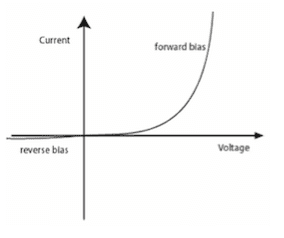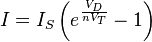# Resistance of a diode

Hello,
A diode is represented by an EMF equal to the electric field in depletion zone plus a resistance. This resistance is obtained from the I/U characteristic of a diode. My question is, this resistance is due to what? The resistivity of P and N junctions or because in forward bias case, there's still a depletion region (electric field) that resists the flow of charges? I know in both case we have an electric field, but in both case is there heat dissipation?

Thank you.

Last edited:

Henryk
Gold Member
I don't think that I ever encountered modeling a diode as an EMF plus resistance. Diode is a non-linear element and the usual definition of resistance (i.e. R = V/I) does not apply. You can define a differential resistance (## \frac 1 R = \frac {dI} {dV} ##) which is the resistance the diode present to a very small signal. There is also a non-linear capacitance in parallel with the junction resistance. Another thing, the junction thickness is very small. There is a resistance of the body of the semiconductor and it is in series with the junction.
Now to answer your question. The resistance is the result of the fact that the energy a carrier (an electron or a hole) acquires due to the field is lost in collision with crystal vibrations, impurities, etc.
And yes, there is heat dissipation, always. The total power input to the diode is equal to V*I. Some of this power can be converted to light, like in LEDs, but the rest is dissipated as heat.

Great. In reverse bias mode, the diode has a small current (saturation current). The reverse saturation current is due to the diffusive flow of minority electrons from the p-side to the n-side and the minority holes from the n-side to the p-side. When the diffusion current is crossing the depletion region that has a voltage Vd, there's a loss of power equal to Vd x Io right? but also flowing through the depletion region, this depletion region has a very low conductivity thus a very high resistivity, so how this resistivity can be modelled? What it is confusing me, that in the depletion region we have an electric field due to charge separation and this depletion region has a conductivity thus resistance

Plus what is confusing me, that diffusion current is due to difference of charge concentration, but while flowing through depletion region, that has an electric field thus voltage drop, this depletion region also has a conductivity. So how this conductivity affects the diffusion current? And this conductivity or resistance does not also cause a potential drop?

sophiecentaur
Gold Member
2020 Award
this depletion region also has a conductivity

The model you started with (i.e. a depletion region plus a resistor) is only an Equivalent Circuit. Just as a real battery is described as a voltage source in series with an internal resistance but that resistance is not actually sited in series with the other component. The resistance in the diode can be partly or totally in the depletion region but you can't separate them out physically and measure just one.

•tonyjk
Henryk
Gold Member
You can't really use the concept of resistance for a diode. Depletion layer is really void of carriers. The electric field there sweeps them away. The reason that reversly-polarized diode carries current is generation of electron and holes there. The current is really equal to the total number of electron and holes generated per unit time within the depletion layer. In the forward polarization, you have to apply the voltage across the junction roughly equal to the height of the potential barrier to start seeing any appreciable field.

•tonyjk
You can't really use the concept of resistance for a diode. Depletion layer is really void of carriers. The electric field there sweeps them away. The reason that reversly-polarized diode carries current is generation of electron and holes there. The current is really equal to the total number of electron and holes generated per unit time within the depletion layer. In the forward polarization, you have to apply the voltage across the junction roughly equal to the height of the potential barrier to start seeing any appreciable field.
Ok but during their sweep in the depletion region, they do not encounter a resistance to flow due to conductivity in the reverse bias mode? If yes, how it is modeled? Can we say, it is mentioned implicitly in the value of this current?

Another question:
In the case of forward biased pn junction, the barrier potential across pn-junction = Vbi-Vf. Using this equation, how can we explain the case if Vf > Vbi?When taking this graph which illustrates an ideal diode (without the resistance of quasi-neutral region (P and N), when the applied voltage is more than the potential barrier (for example 0.7V), then the potential barrier disappear? Then what is the resistance here? of what? If it is of the P and N region, it is false because this is the characteristic of an ideal diode without the quasi-neutral region resistance. So, this resistance represents what?

Last edited:
CWatters
Homework Helper
Gold Member
At the end of the day all the model is trying to do is approximate a real diode IV curve. Without resistance in the model the current would be infinite once 0.7V is exceeded. It's not trying to model the "resistance" of a real diode with any great accuracy as that isn't possible with such a simple model. The slope (aka resistance) of a real diode isn't constant.

Without resistance in the model..
With resistance..

Last edited by a moderator:This equation is for a diode without the resistance of the quasi-neutral region. This equation is used to plot the characteristic of the diode that I mentioned in my post #8. In this equation, we are calculating the resistance and it is equal to V/I for V> 0.7V for example. But this resistance is of what? Considered that this equation does not take into consideration the resistance of the P and N junction.

Henryk
Gold Member
My apology, I stated in my previous post '.. appreciable field' but I mean to say '...appreciable current'

For most semiconductors, the electrons obey Botzmann statistics, that is probability of finding electron of energy ##\mathcal E## is proportional to
## p(\mathcal E ) \sim exp(- \frac {\mathcal E}{kT}) ##. The total electron energy is its band energy plus its kinetic energy plus any potential energy due to electric field. So, if within the bulk of the n-region the concentration of electron is ##n_0##, in the depletion region, there is an extra potential energy equal -eV(r) added that reduces the number of electrons to ##n(r) = n_0 exp( \frac {-eV}{kT})##
This leads directly to the Schottky equation for the diode: the forward current vs voltage characteristics simply reflects the number of electrons that have enough energy to cross over the potential barrier of the junction.
Next thing, is the total current density is ## j = e j_p - e j_n## where ## j_p## and ## j_n## are hole and electron fluxes.
The equation for, say electron flux is ##j_n = -e \mu _n \vec E - D\nabla n##. The first term is the drift due to electric field and the second is diffusional flux.
In the depletion region with no voltage applied to the junction, we do have electric field but no net current because the drift and diffusional fluxes cancel out exactly.
They have to because otherwise, we would be violating the second law of thermodynamics.
These are the basic equations. With these, you can derive the diode equation and all the details of its operation.
I hope it will help to clear the confusion.

•tonyjk